## 1. 拉格朗日乘子法

### 1.1 定义

$$L(x_1,x_2, …, x_n, λ_1,λ_2,…,λ_n)=f(x_1,x_2, …, x_n) - \sum_{i=1}^{k}λ_ig_i(x_1,x_2, …, x_n)$$

## 2. 等式约束条件的优化问题

### 2.1 解法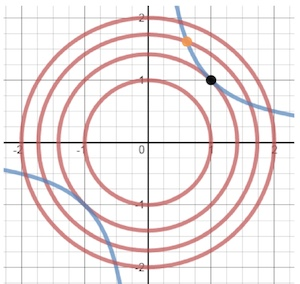$\frac{∂f}{∂x}=λ\frac{∂g}{∂x}$
$\frac{∂f}{∂y}=λ\frac{∂g}{∂y}$
$g(x,y)=0$

$\frac{∂L}{∂x}=\frac{∂f}{∂x}−λ\frac{∂g}{∂x}=0$
$\frac{∂L}{∂y}=\frac{∂f}{∂y}−λ\frac{∂g}{∂y}=0$
$\frac{∂L}{∂λ}=g(x,y)=0$

$L(x_1,…,x_n,λ_1,…,λ_k)=f(x1,…,xn)−\sum_{j=1}^{k}λ_jg_j(x_1,…,x_n)$

### 2.2 进一步理解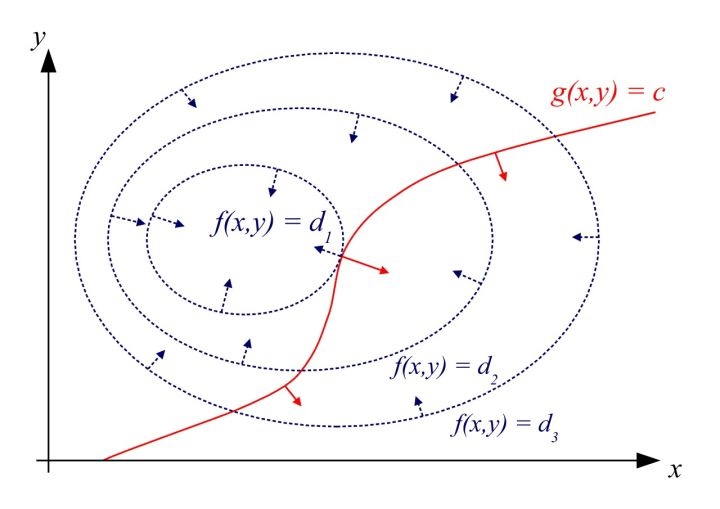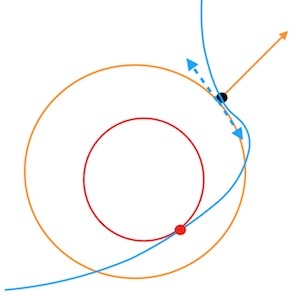## 3. 凸优化

### 3.1 凸优化的意义

1. 其应用非常广泛，机器学习中很多优化问题都要通过凸优化来求解；
2. 在非凸优化中，凸优化同样起到重要的作用，很多非凸优化问题，可以转化为凸优化问题来解决；
3. 如上引用所述，凸优化问题可以看作是具有成熟求解方法的问题，而其他优化问题则未必。

### 3.3 对偶问题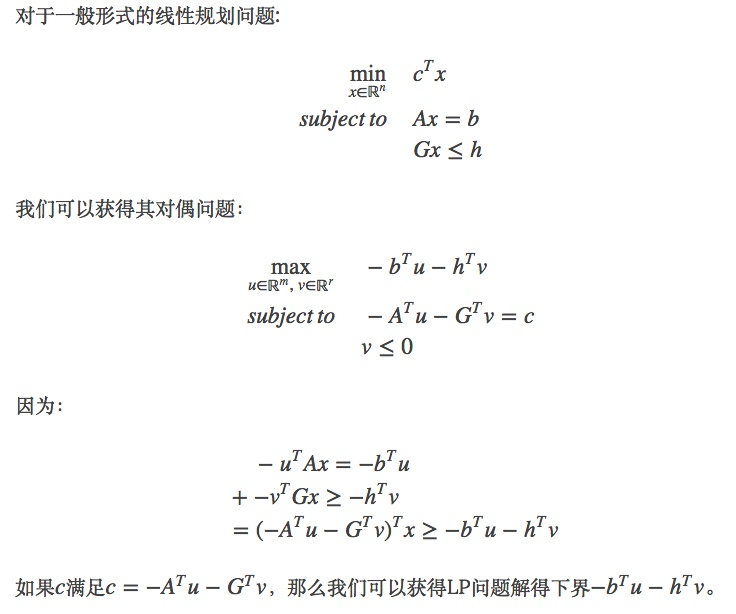## 4. 不等式约束条件的优化问题

### 4.1 广义拉格朗日函数

$min.:f(x)$
$s.t.:$
$g_i(x) ≤ 0, i=1,2,…,p,$
$h_j(x) = 0, j=1,2,…,q,$
$x∈Ω⊂Rn$

$$L(x,λ,μ)=f(x)+\sum_{i=1}^{p}μ_ig_i(x)+\sum_{j=1}^{q}λ_jh_j(x)$$

### 4.2 定义原始问题最优解

$$\theta_p(x)={max}_{λ,μ:μ_i\ge0}L(x,λ,μ)=L(x,λ,μ)$$

1. 如果 $x$ 违反了约束条件，即 $g_i(x) > 0$ 或者 $h_j(x) \neq 0$，则容易存在 $μ_i -> +∞$，也容易存在 $λ_j$ 使得 $λ_jh_j(x) -> +∞$，这样显然 $\theta_p(x)$ 是没有最大值的。
2. 如果 $x$ 满足了约束条件，则 $\theta_p(x)={max}_{λ,μ:μ_i\ge0}L(x,λ,μ)={max}_{λ,μ:μ_i\ge0}f(x)=f(x)$，因为 ${max}_{λ,μ:μ_i\ge0}f(x)$ 与 $λ,μ$ 没有关系，所以其等于 $f(x)$。

### 4.3 定义对偶问题

$$\theta_D(λ,μ)={min}_xL(x,λ,μ)$$

### 4.4 对偶问题与原始问题的关系

1. 原始问题是凸优化问题的时候
2. 原始问题满足 Slater 条件（这一条还可以换成其他条件）
3. 最优解满足 KKT 条件

#### Slater 条件

$p^∗=d^∗=L(x^∗,α^∗,β^∗)$

### 4.5 KKT条件

KKT 条件(Karush-Kuhn-Tucker Conditions)，是指在满足一些有规则的条件下, 一个非线性规划(Nonlinear Programming)问题能有最优化解法的一个必要和充分条件，这是一个广义化拉格朗日乘数的成果。$$L(x,λ,μ)=f(x)+\sum_{i=1}^{p}μ_ig_i(x)+\sum_{j=1}^{q}λ_jh_j(x)$$

1. 约束条件满足 $g_i(x^*)≤0,i=1,2,…,p$, 以及 $h_j(x^*)=0,j=1,2,…,q$
2. $∇f(x^*)+\sum_{i=1}^{p}μ_i∇g_i(x^*)+\sum_{j=1}^{q}λ_j∇h_j(x^*)=0$, 其中$∇$为梯度算子，也就是 L(x,u,λ) 对 x 求导为 0
3. $λ_j≠0$ 且不等式约束条件满足 $μ_i≥0$, $μ_ig_i(x^*)=0,i=1,2,…,p$，因为 $g(x)<=0$，如果要满足这个等式，必须 $μ_i=0$ 或者 $g(x)=0$

#### KKT条件的推导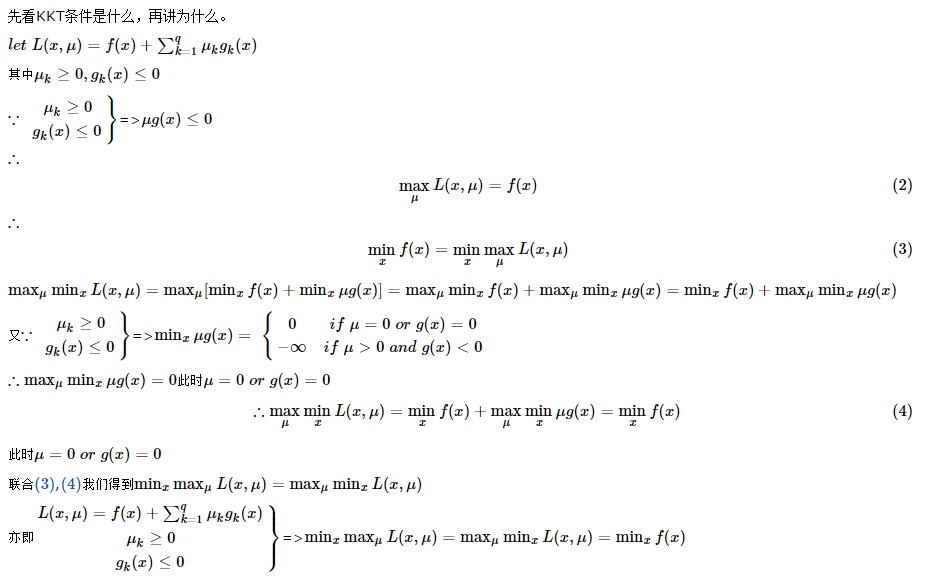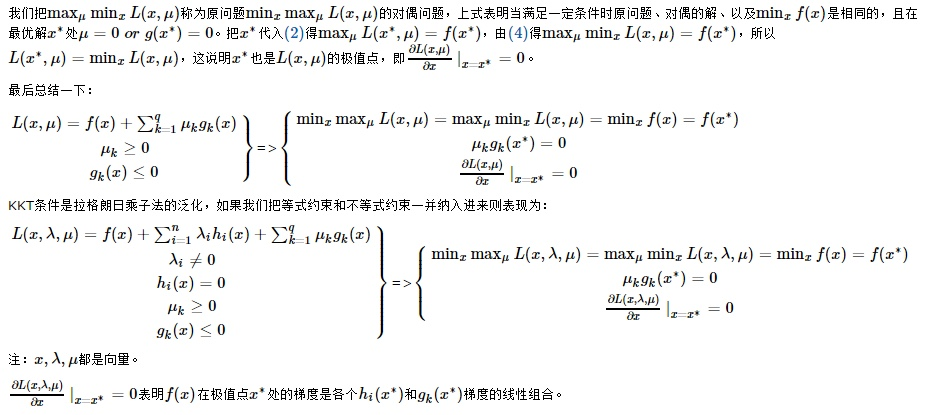### 4.6 总结

• 原问题是一个凸优化问题，则可以直接应用 KKT 条件来求解
• 原问题不是一个凸优化问题，则通过对偶问题转化为凸优化问题，在满足强对偶的条件下应用 KKT 条件求解